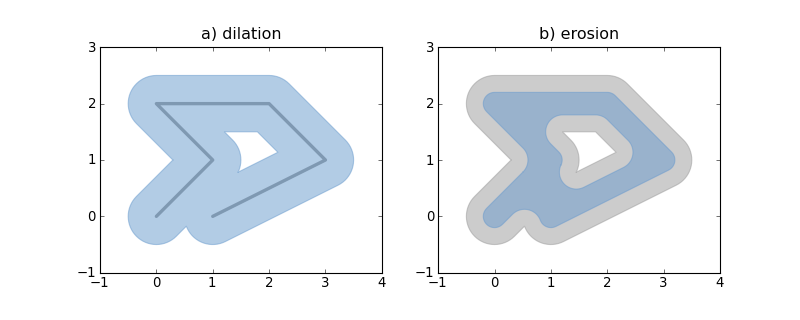Use geometric objects as matplotlib paths and patches

## Project description

Use Shapely or GeoJSON-like geometric objects as matplotlib paths and patchesRequires: matplotlib, numpy, and optionally Shapely 1.2+.

Example:

from matplotlib import pyplot
from shapely.geometry import LineString
from descartes import PolygonPatch

BLUE = '#6699cc'
GRAY = '#999999'

def plot_line(ax, ob):
x, y = ob.xy
ax.plot(x, y, color=GRAY, linewidth=3, solid_capstyle='round', zorder=1)

line = LineString([(0, 0), (1, 1), (0, 2), (2, 2), (3, 1), (1, 0)])

fig = pyplot.figure(1, figsize=(10, 4), dpi=180)

# 1

plot_line(ax, line)

dilated = line.buffer(0.5)
patch1 = PolygonPatch(dilated, fc=BLUE, ec=BLUE, alpha=0.5, zorder=2)

#2

patch2a = PolygonPatch(dilated, fc=GRAY, ec=GRAY, alpha=0.5, zorder=1)

eroded = dilated.buffer(-0.3)

# GeoJSON-like data works as well

polygon = eroded.__geo_interface__
# >>> geo['type']
# 'Polygon'
# >>> geo['coordinates'][:2]
# ((0.50502525316941682, 0.78786796564403572), (0.5247963548222736, 0.8096820147509064))
patch2b = PolygonPatch(polygon, fc=BLUE, ec=BLUE, alpha=0.5, zorder=2)

pyplot.show()

Descartes is not associated with the identically named and apparently defunct project at http://descartes.sourceforge.net/.

## Project details1.1.0

This version1.0.21.0.11.00.1.20.1.10.1

### Source Distribution

descartes-1.0.2.tar.gz (3.3 kB view hashes)

Uploaded source

### Built Distributions

descartes-1.0.2-py3-none-any.whl (5.6 kB view hashes)

Uploaded py3

descartes-1.0.2-py2-none-any.whl (5.6 kB view hashes)

Uploaded py2